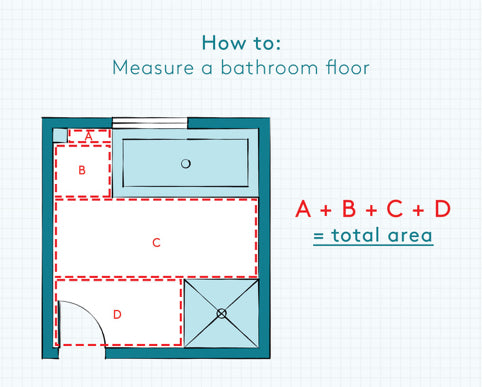# How To Measure Your Room For Tiles

We’re often asked to help work out how many tiles a customer will need to order for their latest project and it’s no problem at all. We’re the experts after all!

The thing is, it’s not a particularly difficult thing to do yourself. With a bit of guidance you’ll quickly be able to calculate what you need and don’t worry, a maths degree isn’t required! But of course, if in doubt, just pick up the phone and give us a call. We’ll be more than happy to help.

The basic method for calculating the area of floors and walls is the same, but there’s a few things you’ll need to watch out for so we’ll take a look at them separately in this guide.

### Here’s what you’ll need to get cracking:

1 A tape measure
2 A Notepad & a pencil or pen
3 A calculator
4 A willing and able assistant (although proper DIY’ers can fly solo)
5 Patience (it’s a virtue remember)
6 A builders brew (you’re doing DIY, it’s allowed)

It’s best to work in centimetres to begin. Inches and feet are allowed of course, but you’ll find that most tile shops and tilers prefer to work in the decimal system which is millimetres (mm), centimetres (cm) and metres (m).

So let’s start with the easiest of all room shapes… a straightforward rectangle, like below.1 Measure wall A and note down measurement in cm, e.g. 260 cm
2 Measure wall B and note down measurement in cm, e.g. 400 cm
3 To work out the area, take you calculator and multiply Wall A x Wall b

260 x 400 = 104000 cm2

4. We now need to convert this into square metres (m2)
To do this we must divide by 10000

5. So…

104000/10000 = 10.40m2

2 decimal places is enough to round up or down to.
Now you have the exact area in m2.

6. We now need to add in an allowance for wastage.
Most of the time, 10% extra will be enough to cover you for wastage and cuts
There’s a quick way to add 10% to your area which is to multiply it by 1.1

10.4 x 1.1 = 11.44m2

This is the amount of tiles in square metres you’ll need.

Next up, a more complicated room shape. Think rooms with pipes boxed in, or baths on the floor.

Let’s look at a room complete with things that get in the way, e.g. your bathroom.This kind of room requires a bit more effort but it’s not to difficult if you follow the steps below:

1 Start with sketching a plan view of the room like I’ve down below. Don’t worry, no one will judge your drawing skills, you’re just doing this to work things out!

2 Divide your sketch up into regular rectangle shapes, making sure you don’t miss out any areas. Give your rectangles names. A, B, C… etc,

3 Now it’s simply a case of working out the area of each named rectangle and dividing by 1000 to get the area in square metres (m2). So…

A 123 x 123 cm = 15129cm2 = 1.51m2
B 226 x 250 cm = 56500cm2 = 5.65m2
C
D

4. Now add up all these areas and hey presto, you have a total.

A + B + C + D =

5. Hopefully the eagled eyed amongst you will have realised that we need to now allow for 10% wastage. And how do we do that? Remember the simple calculation….

Total * 1.1 = Total including 10% wastage. So…

19.56 * 1.1 = 21.80m2

Give yourself (and your assistant) a pat on the back then have a swig of your ever so sweet tea. You’ve earned it. You can now calculate the area of a floor. Next up, walls.

Walls are generally rectangular shaped too, but sometimes they have windows or doors on them that need to be accounted for.

So what we need to do is first calculate the area of the entire wall, then subtract the area of the window or door that’s also on the wall. It goes without saying that you wouldn’t want to tile over your window.

Let’s begin. You only need to measure the bit of wall that you want to tile. In this example below, we’re going to be tiling the splash back in the kitchen. Again it’s a good idea to do a quick sketch of the wall in question.

1. Measure the length of the wall area you want to tile
2. Measure the height of the wall area you want to tile
3. Calculate the area of the wall (Length x height)

So 245 x 250 = 61250 = 6.13m2

4. Now measure the window that’s on the wall

So 120 x 120 = 14400 = 1.44 m2

5. Now you need to subtract the area of the window from the wall.

6.13 – 1.44 = 4.69 m2

6. And finally, we need to add the 10% wastage onto our total using the handy calculation again:

Total x 1.1 = Total including 10% wastage

4.69 x 1.1 = 5.16 m2

If there are other walls you need to tile you’ll need to do the same for each of these and then add each of the totals together.

Hopefully this guide will have given you a pretty good idea of how to calculate how many tiles you’ll need to order for your next project. If you need any help at all measuring up or working things out, just give us a call or drop us an email. We’re here to help!